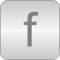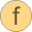# Functions

Functions for multi phase systems# Package ContentsquasiRMS Calculate continuous quasi RMS value of inputactivePower Calculate active power of voltage and current inputsymmetricOrientation Orientations of the resulting fundamental wave field phasorssymmetricOrientationMatrix Matrix symmetric orientation angles for creating the symmetric transformation matrixsymmetricTransformationMatrix Transformation matrix for symmetrical componentssymmetricBackTransformationMatrix Back transformation matrix for symmetrical componentsnumberOfSymmetricBaseSystems Determines the number of symmetric base systems of m phase symmetric systemfactorY2D Calculates factor Y voltage to polygon (delta) voltagefactorY2DC Calculates factor of DC-voltage from RMS Y-voltageindexPositiveSequence Determines the indices of the all positive sequencesindexNonPositiveSequence Determines the indices of all non positive sequences